×
Get Full Access to College Physics - 1 Edition - Chapter 10 - Problem 1cq
Get Full Access to College Physics - 1 Edition - Chapter 10 - Problem 1cq

×

# Analogies exist between rotational and translationalISBN: 9781938168000 42

## Solution for problem 1CQ Chapter 10

College Physics | 1st Edition

• Textbook Solutions
• 2901 Step-by-step solutions solved by professors and subject experts
• Get 24/7 help from StudySoup virtual teaching assistantsCollege Physics | 1st Edition

4 5 1 394 Reviews
30
0
Problem 1CQ

Problem 1CQ

Analogies exist between rotational and translational physical quantities. Identify the rotational term analogous to each of the following: acceleration, force, mass, work, translational kinetic energy, linear momentum, impulse.

Step-by-Step Solution:

Q:Analogies exist between rotational and translational physical quantities. Identify the rotational term analogous to each of the following: acceleration, force, mass, work, translational kinetic energy, linear momentum, impulse.

Step By Step Solution

Step 1 of 2

(1) Acceleration : The analogue for linear acceleration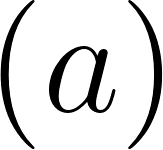in rotational motion is the angular acceleration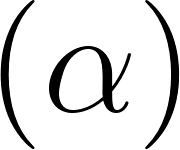. The S.I unit of linear acceleration is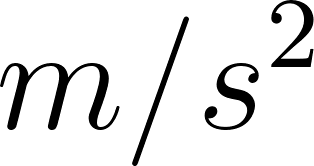whereas the S.I unit of angular acceleration is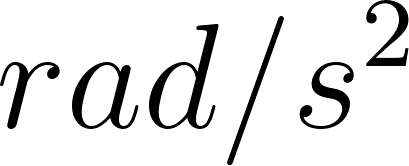.

(2) Force : Forceis the product of mass and the linear acceleration. Torque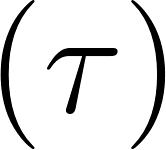is the analogue for force in rotational motion. Torque is the product of moment of inertia and angular acceleration. The S.I unit of Force is Newton, whereas the S.I unit of torque is Newton-meter.

(3) Mass : In rotational moment of inertiais the analogue to the mass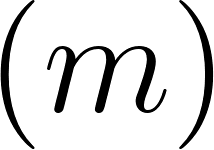in linear motion. The moment of inertia is the product of mass of the system of rotating particle and square of the perpendicular distance of particle from rotational axis. Mathematically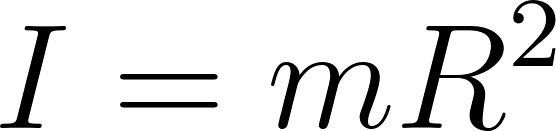. The S.I unit of mass isand the S.I unit of moment of inertia is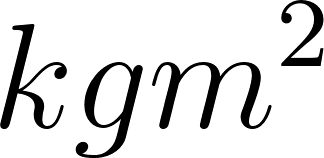.

Step 2 of 2

##### ISBN: 9781938168000

College Physics was written by and is associated to the ISBN: 9781938168000. The answer to “Analogies exist between rotational and translational physical quantities. Identify the rotational term analogous to each of the following: acceleration, force, mass, work, translational kinetic energy, linear momentum, impulse.” is broken down into a number of easy to follow steps, and 28 words. This textbook survival guide was created for the textbook: College Physics , edition: 1. The full step-by-step solution to problem: 1CQ from chapter: 10 was answered by , our top Physics solution expert on 07/07/17, 04:39PM. This full solution covers the following key subjects: rotational, translational, Linear, Energy, exist. This expansive textbook survival guide covers 34 chapters, and 3125 solutions. Since the solution to 1CQ from 10 chapter was answered, more than 1083 students have viewed the full step-by-step answer.

Unlock Textbook Solution